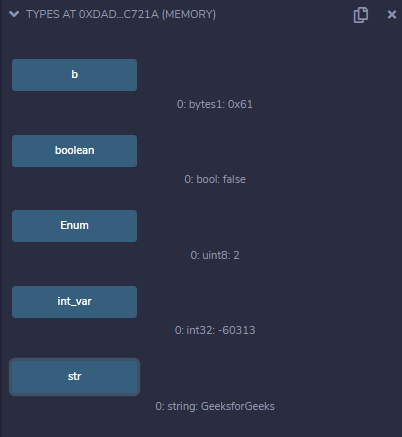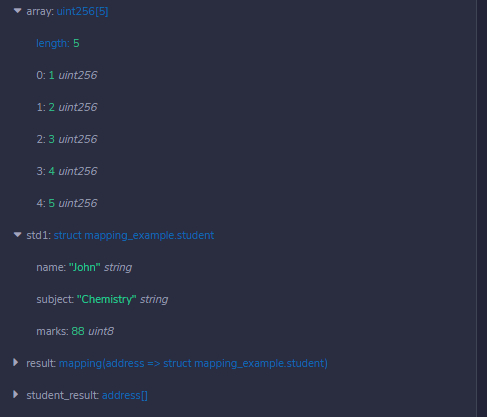Related Articles

# Solidity – Types

Solidity is a statically typed language, which implies that the type of each of the variables should be specified. Data types allow the compiler to check the correct usage of the variables. The declared types have some default values called Zero-State, for example for bool the default value is False. Likewise other statically typed languages Solidity has Value types and Reference types which are defined below:

### Value Types

Value-type variables store their own data. These are the basic data types provided by solidity. These types of variables are always passed by value. The variables are copied wherever they are used in function arguments or assignments. Value type data types in solidity are listed below:

• Boolean: This data type accepts only two values True or False.
• Integer: This data type is used to store integer values, int, and uint are used to declare signed and unsigned integers respectively.
• Fixed Point Numbers: These data types are not fully supported in solidity yet, as per the Solidity documentation. They can be declared as fixed and unfixed for signed and unsigned fixed-point numbers of varying sizes respectively.
• Address: Address hold a 20-byte value which represents the size of an  Ethereum address. An address can be used to get a balance or to transfer a balance by balance and transfer method respectively.
• Bytes: Although bytes are similar to strings, there are some differences between them. bytes used to store a fixed-sized character set while the string is used to store the character set equal to or more than a byte. The length of bytes is from 1 to 32, while the string has a dynamic length. Byte has the advantage that it uses less gas, so better to use when we know the length of data.
• Enums: It is used to create user-defined data types, used to assign a name to an integral constant which makes the contract more readable, maintainable, and less prone to errors. Options of enums can be represented by unsigned integer values starting from 0.

Example:

In the below example, the contract Types initialize the values of different types of Values Types.

## Solidity

 `// SPDX-License-Identifier: GPL-3.0 ` `pragma solidity >=0.4.22 <0.9.0;  ` `/// @title A contract for demonstrate Value types` `/// @author Jitendra Kumar` `/// @notice For now, this contract just show how Value types works in solidity` `contract` `Types {`   `    ``// Initializing Bool variable` `    ``bool` `public` `boolean = ``false``;` `    `  `    ``// Initializing Integer variable` `    ``int32 ``public` `int_var = -60313;`   `    ``// Initializing String variable` `    ``string ``public` `str = ``"GeeksforGeeks"``;`   `    ``// Initializing Byte variable` `    ``bytes1 ``public` `b = ``"a"``;` `    `  `    ``// Defining an enumerator` `    ``enum` `my_enum { geeks_, _for, _geeks }`   `    ``// Defining a function to return` `    ``// values stored in an enumerator` `    ``function Enum() ``public` `pure returns(` `    ``my_enum) {` `        ``return` `my_enum._geeks;` `    ``}` `}`

Output:### Reference Types

Reference type variables store the location of the data. They don’t share the data directly. With the help of reference type, two different variables can refer to the same location where any change in one variable can affect the other one. Reference types in solidity are listed below:

• Arrays: An array is a group of variables of the same data type in which the variable has a particular location known as an index. By using the index location, the desired variable can be accessed. The array size can be fixed or dynamic.
• Strings: Strings are like arrays of characters. When we use them, we might occupy bigger or shorter storage space.
• Struct: Solidity allows users to create and define their own type in the form of structures. The structure is a group of different types even though it’s not possible to contain a member of its own type. The structure is a reference type variable that can contain both value type and reference type
• Mapping: Mapping is the most used reference type, that stores the data in a key-value pair where a key can be any value type. It is like a hash table or dictionary as in any other programming language, where data can be retrieved by key.

Example: In the below example, the contract Types initialize the values of various Reference Types.

## Solidity

 `// SPDX-License-Identifier: GPL-3.0 ` `pragma solidity >=0.4.22 <0.9.0;  ` `/// @title A contract for demonstrate Reference types` `/// @author Jitendra Kumar` `/// @notice For now, this contract just show how Reference types works in solidity` `contract` `mapping_example {`   `    ``// Defining an array` `    ``uint ``public` `array` `    ``= [uint(1), 2, 3, 4, 5] ;` `    `  `    ``// Defining a Structure` `    ``struct` `student {` `        ``string name;` `        ``string subject;` `        ``uint8 marks;` `    ``}`   `    ``// Creating a structure object` `    ``student ``public` `std1;`   `    ``// Defining a function to return` `    ``// values of the elements of the structure` `    ``function structure() ``public` `returns(` `    ``string memory, string memory, uint){` `        ``std1.name = ``"John"``;` `        ``std1.subject = ``"Chemistry"``;` `        ``std1.marks = 88;` `        ``return` `(` `        ``std1.name, std1.subject, std1.marks);` `    ``}` `    `  `    ``// Creating a mapping` `    ``mapping (address => student) result;` `    ``address[] student_result;` `}`

Output:Whether you're preparing for your first job interview or aiming to upskill in this ever-evolving tech landscape, GeeksforGeeks Courses are your key to success. We provide top-quality content at affordable prices, all geared towards accelerating your growth in a time-bound manner. Join the millions we've already empowered, and we're here to do the same for you. Don't miss out - check it out now!